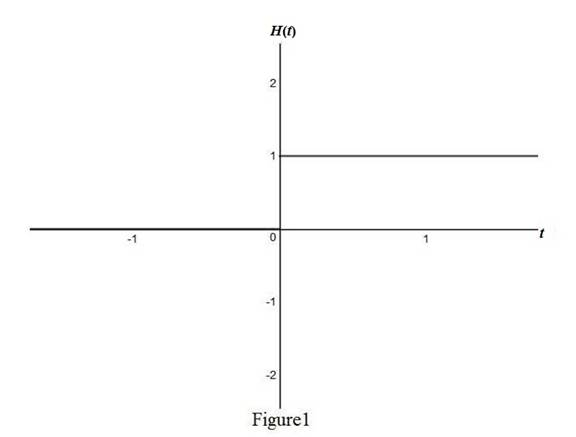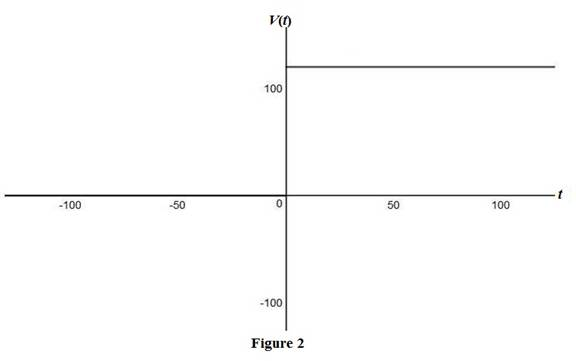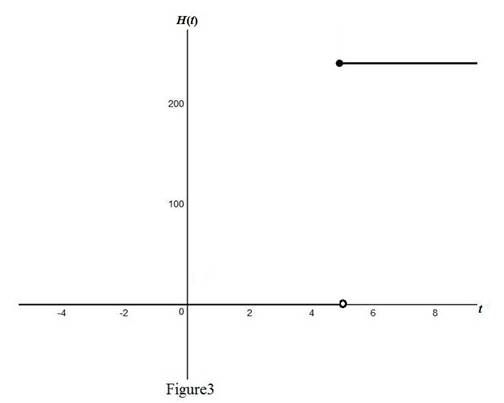# The graph of the Heaviside function.### Single Variable Calculus: Concepts...

4th Edition
James Stewart
Publisher: Cengage Learning
ISBN: 9781337687805### Single Variable Calculus: Concepts...

4th Edition
James Stewart
Publisher: Cengage Learning
ISBN: 9781337687805

#### Solutions

Chapter 1.3, Problem 57E

(a)

To determine

## To sketch: The graph of the Heaviside function.

Expert Solution

### Explanation of Solution

The Heaviside function is, H(t)={0      if t<01if t0.

Use online graphing calculator and draw the graph of the Heaviside function as shown below in Figure1.From Figure1, it is observed the Heaviside function is a piece-wise continuous function.

(b)

To determine

### To sketch: The graph of V(t) in a circuit if the switch is turned on at time t = 0 and 120 volts are applied to the circuit; write the formula for V(t) in terms of H(t).

Expert Solution

The formula for V(t) in terms of H(t) is V(t)=120H(t).

### Explanation of Solution

Observe that when t<0, the voltage is 0 and when t0, the voltage is 120.

Thus, the formula for V(t) can be written in form of H(t) as, V(t)={0          if t<0120if t0.

Use online graphing calculator and draw the graph of V(t)={0          if t<0120if t0 as shown below in Figure 2.From Figure2, it is observed that the voltage is 0 when t = 0.

(c)

To determine

Expert Solution

### Explanation of Solution

Given that the time is t = 5 seconds.

Express the time as, t5=0.

Thus, the formula for V(t) after t=5s is V(t)=240H(t5).

Use online graph calculator and draw the graph of the voltage V(t)=240H(t5) as shown below in Figure 3.From Figure 3, notice that the number 5 is not included in the domain as the switch is turned on at t =  5 seconds.

### Have a homework question?

Subscribe to bartleby learn! Ask subject matter experts 30 homework questions each month. Plus, you’ll have access to millions of step-by-step textbook answers!